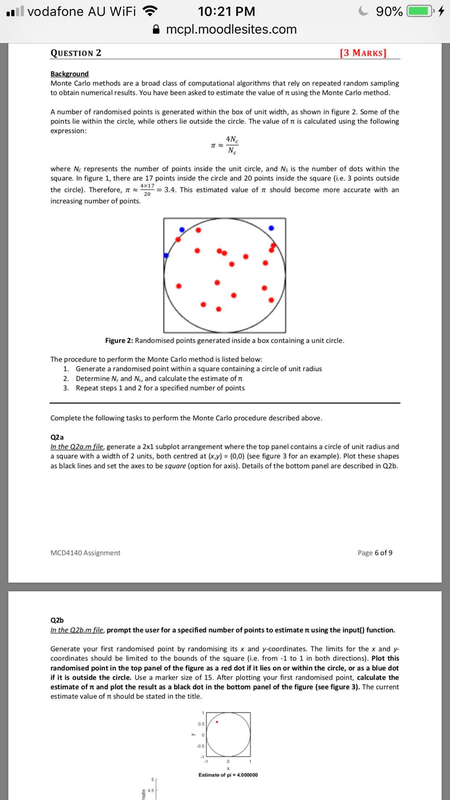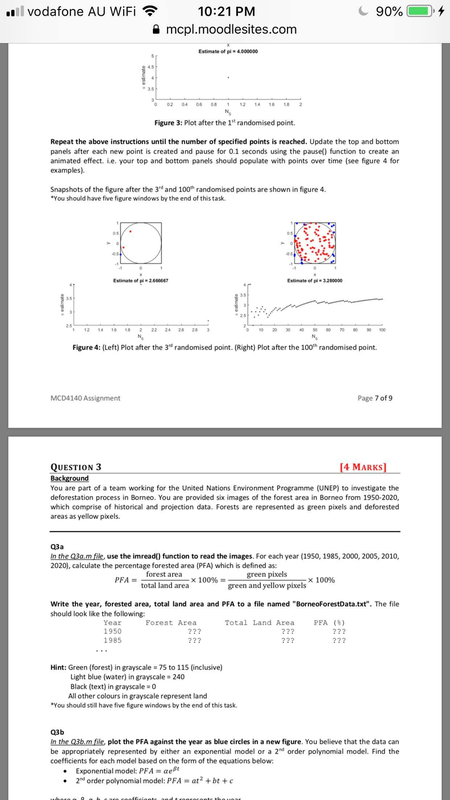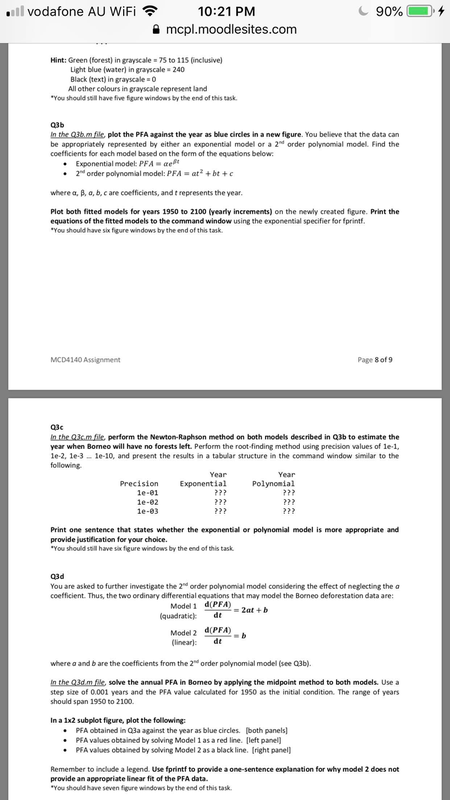# We Helped With This Machine Learning Assignment: Have A Similar One?

SOLVEDCategory Math Machine Learning College Solved Applied Data Science With Python Assignment

## Assignment Image.vodafone AU WiFi QUESTION 2 10:21 PM mcpl.moodlesites.com Background Monte Carlo methods are a broad class of computational algorithms that rely on repeated random sampling to obtain numerical results. You have been asked to estimate the value of using the Monte Carlo method. A number of randomised points is generated within the box of unit width, as shown in figure 2. Some of the points lie within the circle, while others lie outside the circle. The value of it is calculated using the following expression: where Nc represents the number of points inside the unit circle, and Ns is the number of dots within the square. In figure 1, there are 17 points inside the circle and 20 points inside the square (i.e. 3 points outside the circle). Therefore, = 3.4. This estimated value of should become more accurate with an increasing number of points. 4x17 20 Figure 2: Randomised points generated inside a box containing a unit circle. The procedure to perform the Monte Carlo method is listed below: 1. Generate a randomised point within a square containing a circle of unit radius 2. Determine Nc and Ns, and calculate the estimate of 3. Repeat steps 1 and 2 for a specified number of points MCD4140 Assignment Complete the following tasks to perform the Monte Carlo procedure described above. T≈ 4Nc N 4.5 Q2a In the Q2a.m file, generate a 2x1 subplot arrangement where the top panel contains a circle of unit radius and a square with a width of 2 units, both centred at (x,y) = (0,0) (see figure 3 for an example). Plot these shapes as black lines and the axes to be square (option for axis). Details of the bottom panel are described in Q2b. 0.5 0 90% [3 MARKS] Q2b In the Q2b.m file, prompt the user for a specified number of points to estimate using the input() function. -0.5 Generate your first randomised point by randomising its x and y-coordinates. The limits for the x and y- coordinates should be limited to the bounds of the square (i.e. from 1 to 1 in both directions). Plot this randomised point in the top panel of the figure as a red dot if it lies on or within the circle, or as a blue dot if it is outside the circle. Use a marker size of 15. After plotting your first randomised point, calculate the estimate of π and plot the result as a black dot in the bottom panel of the figure (see figure 3). The current estimate value of it should be stated in the title. -1 0 X Estimate of pi= 4.000000 1 Page 6 of 9

## Assignment Image.vodafone AU WiFi estimate 2.5 0.5 0 -0.5 -1 12 14 1.6 MCD4140 Assignment ● whorn 4 Repeat the above instructions until the number of specified points is reached. Update the top and bottom panels after each new point is created and pause for 0.1 seconds using the pause() function to create an animated effect. i.e. your top and bottom panels should populate with points over time (see figure 4 for examples). Snapshots of the figure after the 3rd and 100th randomised points are shown in figure 4. *You should have five figure windows by the end of this task. 3.5 0 1.8 3 10:21 PM mcpl.moodlesites.com 0 Year 1950 1985 1 Ng Figure 3: Plot after the 1st randomised point. Estimate of pi = 2.666667 X Estimate of pi= 4.000000 02 0.4 0.6 0.8 3 1.2 Forest Area ??? ??? Hint: Green (forest) in grayscale = 75 to 115 (inclusive) Light blue (water) in grayscale = 240 1.4 1.6 1.8 2.5 Black (text) in grayscale = 0 All other colours in grayscale represent land *You should still have five figure windows by the end of this task. 2 22 2.4 2.6 2.8 N₂ Figure 4: (Left) Plot after the 3rd randomised point. (Right) Plot after the 100th randomised point. 10 2 20 Estimate of pi = 3.280000 QUESTION 3 [4 MARKS] Background You are part of a team working for the United Nations Environment Programme (UNEP) to investigate the deforestation process in Borneo. You are provided six images of the forest area in Borneo from 1950-2020, which comprise of historical and projection data. Forests are represented as green pixels and deforested areas as yellow pixels. 30 40 Q3a In the Q3a.m file, use the imread() function to read the images. For each year (1950, 1985, 2000, 2005, 2010, 2020), calculate the percentage forested area (PFA) which is defined as: forest area PFA = x 100% = total land area green pixels green and yellow pixels are coofficients and troprosents the wor 50 60 70 80 N₂ Write the year, forested area, total land area and PFA to a file named "BorneoForestData.txt". The file should look like the following: Total Land Area ??? ??? 90% 90 100 X 100% Page 7 of 9 PFA (%) ??? ??? Q3b In the Q3b.m file, plot the PFA against the year as blue circles in a new figure. You believe that the data can be appropriately represented by either an exponential model or a 2nd order polynomial model. Find the coefficients for each model based on the form of the equations below: ● Exponential model: PFA = aeßt 2nd order polynomial model: PFA = at² + bt + c 4

## Assignment Imagel vodafone AU WiFi Hint: Green (forest) in grayscale = 75 to 115 (inclusive) Light blue (water) in grayscale = 240 Black (text) in grayscale = 0 All other colours in grayscale represent land *You should still have five figure windows by the end of this task. MCD4140 Assignment Q3b In the Q3b.m file, plot the PFA against the year as blue circles in a new figure. You believe that the data can be appropriately represented by either an exponential model or a 2nd order polynomial model. Find the coefficients for each model based on the form of the equations below: • Exponential model: PFA = αeßt ● 2nd order polynomial model: PFA = at² + bt + c where a, b, a, b, c are coefficients, and t represents the year. Plot both fitted models for years 1950 to 2100 (yearly increments) on the newly created figure. Print the equations of the fitted models to the command window using the exponential specifier for fprintf. *You should have six figure windows by the end of this task. 10:21 PM mcpl.moodlesites.com Q3c In the Q3c.m file, perform the Newton-Raphson method on both models described in Q3b to estimate the year when Borneo will have no forests left. Perform the root-finding method using precision values of 1e-1, 1e-2, 1e-3 ... 1e-10, and present the results in a tabular structure in the command window similar to the following. Precision 1e-01 1e-02 1e-03 ● ● Exponential ● Year In a 1x2 subplot figure, plot the following: Model 2 (linear): ??? ??? ??? Print one sentence that states whether the exponential or polynomial model is more appropriate and provide justification for your choice. *You should still have six figure windows by the end of this task. Q3d You are asked to further investigate the 2nd order polynomial model considering the effect of neglecting the a coefficient. Thus, the two ordinary differential equations that may model the Borneo deforestation data are: Model 1 d(PFA) = 2at + b (quadratic): dt Year Polynomial d(PFA) dt 90% ??? ??? ??? = b where a and b are the coefficients from the 2nd order polynomial model (see Q3b). In the Q3d.m file, solve the annual PFA in Borneo by applying the midpoint method to both models. Use a step size of 0.001 years and the PFA value calculated for 1950 as the initial condition. The range of years should span 1950 to 2100. Page 8 of 9 PFA obtained in Q3a against the year as blue circles. [both panels] PFA values obtained by solving Model 1 as a red line. [left panel] PFA values obtained by solving Model 2 as a black line. [right panel] Remember to include a legend. Use fprintf to provide a one-sentence explanation for why model 2 does not provide an appropriate linear fit of the PFA data. *You should have seven figure windows by the end of this task. 4

Is it free to get my assignment evaluated?

Yes. No hidden fees. You pay for the solution only, and all the explanations about how to run it are included in the price. It takes up to 24 hours to get a quote from an expert. In some cases, we can help you faster if an expert is available, but you should always order in advance to avoid the risks. You can place a new order here.

How much does it cost?

The cost depends on many factors: how far away the deadline is, how hard/big the task is, if it is code only or a report, etc. We try to give rough estimates here, but it is just for orientation (in USD):

 Regular homework \$20 - \$150 Advanced homework \$100 - \$300 Group project or a report \$200 - \$500 Mid-term or final project \$200 - \$800 Live exam help \$100 - \$300 Full thesis \$1000 - \$3000

How do I pay?

Credit card or PayPal. You don't need to create/have a Payal account in order to pay by a credit card. Paypal offers you "buyer's protection" in case of any issues.

Why do I need to pay in advance?

We have no way to request money after we send you the solution. PayPal works as a middleman, which protects you in case of any disputes, so you should feel safe paying using PayPal.

Do you do essays?

No, unless it is a data analysis essay or report. This is because essays are very personal and it is easy to see when they are written by another person. This is not the case with math and programming.

Why there are no discounts?

It is because we don't want to lie - in such services no discount can be set in advance because we set the price knowing that there is a discount. For example, if we wanted to ask for \$100, we could tell that the price is \$200 and because you are special, we can do a 50% discount. It is the way all scam websites operate. We set honest prices instead, so there is no need for fake discounts.

Do you do live tutoring?

No, it is simply not how we operate. How often do you meet a great programmer who is also a great speaker? Rarely. It is why we encourage our experts to write down explanations instead of having a live call. It is often enough to get you started - analyzing and running the solutions is a big part of learning.

What happens if I am not satisfied with the solution?

Another expert will review the task, and if your claim is reasonable - we refund the payment and often block the freelancer from our platform. Because we are so harsh with our experts - the ones working with us are very trustworthy to deliver high-quality assignment solutions on time.

## Popular Solved Assignments Like This

Customer Feedback

"Thanks for explanations after the assignment was already completed... Emily is such a nice tutor! "

Order #13073